# Tension in a Massive Rotating Rope with an Object

• MichaelTam
In summary, the conversation is discussing the problem of finding the tension in a uniform rope attached to a rotating shaft and a point-like object, as a function of the distance from the shaft. The problem assumes that the radius of the shaft is much smaller than the length of the rope and that the effect of gravity can be ignored. The conversation also mentions the use of variables such as m_1, m_2, omega, r, and l to express the answer. Further discussion involves the use of equations for tension and radial acceleration, as well as the concept of integrating over an arbitrary element of the rope. There is also confusion about the relationship between l and r, and the next steps in solving the problem.f

#### MichaelTam

Homework Statement
The question is comes from MIT.
Relevant Equations
The equation are 𝑇(𝑟+Δ𝑟)−𝑇(𝑟)=Δ𝑚𝑎𝑟, find T(r).
The whole question is:
One end of a uniform rope of mass 𝑚1 and length 𝑙 is attached to a shaft that is rotating at constant angular velocity of magnitude 𝜔. The other end is attached to a point-like object of mass 𝑚2. Find 𝑇(𝑟), the tension in the rope as a function of 𝑟, the distance from the shaft.

Assume that the radius of the shaft is much smaller than 𝑙. You may also ignore the effect of gravitation - assume the shaft is rotating fast enough so that the mass and the rope are almost horizontal. Express your answer in terms of some or all of the following variables: m_1 for 𝑚1, m_2 for 𝑚2, omega for 𝜔, 𝑟 and 𝑙.
I can ‘t find the picture only website, you can find the picture at
https://ocw.mit.edu/courses/physics/8-01sc-classical-mechanics-fall-2016/week-4-drag-forces-constraints-and-continuous-systems/problem-set-4/

My misunderstanding is the difference between l and r and the relationship in the question .
𝑟̂ :𝑇(𝑟+Δ𝑟)−𝑇(𝑟)=Δ𝑚𝑎𝑟 where 𝑎𝑟 is the radial acceleration, 𝑎𝑟=−𝜔^2𝑟 . Using that Δ𝑚=𝑚_1/(l)Δ𝑟 , the equation above becomes: 𝑇(𝑟+Δ𝑟)−𝑇(𝑟)=−Δ𝑚𝑚_1*𝑙𝜔^2𝑟. The equation set up byΔ𝑚=𝑚_1/(l)Δ𝑟 is from the tension formula. However this is the point where I don’t understand.I had separate T(r) into T_1 and T_2 , but I don’t know what is the next step?

#### Attachments

•ErnestS and berkeman
Homework Statement:: The question is comes from MIT.
Relevant Equations:: The equation are 𝑇(𝑟+Δ𝑟)−𝑇(𝑟)=Δ𝑚𝑎𝑟, find T(r).

The whole question is:
One end of a uniform rope of mass 𝑚1 and length 𝑙 is attached to a shaft that is rotating at constant angular velocity of magnitude 𝜔. The other end is attached to a point-like object of mass 𝑚2. Find 𝑇(𝑟), the tension in the rope as a function of 𝑟, the distance from the shaft.

Assume that the radius of the shaft is much smaller than 𝑙. You may also ignore the effect of gravitation - assume the shaft is rotating fast enough so that the mass and the rope are almost horizontal. Express your answer in terms of some or all of the following variables: m_1 for 𝑚1, m_2 for 𝑚2, omega for 𝜔, 𝑟 and 𝑙.
I can ‘t find the picture only website, you can find the picture at
https://ocw.mit.edu/courses/physics/8-01sc-classical-mechanics-fall-2016/week-4-drag-forces-constraints-and-continuous-systems/problem-set-4/

My misunderstanding is the difference between l and r and the relationship in the question .
𝑟̂ :𝑇(𝑟+Δ𝑟)−𝑇(𝑟)=Δ𝑚𝑎𝑟 where 𝑎𝑟 is the radial acceleration, 𝑎𝑟=−𝜔^2𝑟 . Using that Δ𝑚=𝑚_1/(l)Δ𝑟 , the equation above becomes: 𝑇(𝑟+Δ𝑟)−𝑇(𝑟)=−Δ𝑚𝑚_1*𝑙𝜔^2𝑟. The equation set up byΔ𝑚=𝑚_1/(l)Δ𝑟 is from the tension formula. However this is the point where I don’t understand.I had separate T(r) into T_1 and T_2 , but I don’t know what is the next step?
L is the distance from the shaft axis to the mass.
r is some arbitrary distance along the rope from the shaft axis, 0<r<L.

You mean both are from centre to centre of the object?And how we define the arbitrary distance, because i still get confused on that meaning.So does the r is not have a fixed length is it?And the L is the length from the shaft axis to the centre of the mass?

the r is not have a fixed length is it?
Right. In order to do the integration, you are considering an arbitrary element of the rope of length dr and distance r from the axis. In the integration, r will range from 0 to L.
L is the length from the shaft axis to the centre of the mass?
Yes.

So , in order to find the T(r), I need to define the range of delta(r) should be 0 to L , and use
T*(r+delta(r))-T(r)=delta(m)*a*r, the staff the tells me delta(m)=m_1/(L*delta(r)) ,but I still don’t know how he can get this result?
So in the solution there are these few lines: 𝑟̂ :𝑇(𝑟+Δ𝑟)−𝑇(𝑟)=Δ𝑚𝑎𝑟 where 𝑎𝑟 is the radial acceleration, 𝑎𝑟=−𝜔^2𝑟 . Using that Δ𝑚=𝑚_1/(l)Δ𝑟 ,
the equation above becomes: 𝑇(𝑟+Δ𝑟)−𝑇(𝑟)=−Δ𝑚
𝑚_1*𝑙𝜔^2𝑟

In the final line, since substitution is done for Δ𝑚, shouldn't Δ𝑚 not be in the equation?

He just said these things to help me, but, it is delta(r) should replace all delta(m) of the upper equations?

ok, now I need to to consider two system , one is the m_1 and second is the m_2 , and I divided the tension acting on them by -T_1 and T_2, and the T_2 should be m_2*omega^2*l because the centripetal force should be m*omega^2*(radius) in this case, so ,what is the next step, I start feel confused.I am confused about the Tension acting by m_2 is how to work as and the inward tension work as.It is
-m_1*omega^2*r+m_2*a*r+m_2*omega^2*r...I think it is a little bit complicated, I can’t put my concept together.

Last edited:
T*(r+delta(r))-T(r)=delta(m)*a*r
No. You seem to be misreading this:
𝑇(𝑟+Δ𝑟)−𝑇(𝑟)=Δ𝑚𝑎𝑟 where 𝑎𝑟 is the radial acceleration, 𝑎𝑟=−𝜔^2𝑟
There are two things wrong or misleading about that. First, it should use dr, not Δr, but I don't want to get sidetracked into that right now. Secondly, ar would be better written as ar.
To make that a bit clearer:
##T(r+dr)-T(r)=a_r.dm ## where ##a_r=-\omega^2r##.
Do you see why that is true?

delta(m)=m_1/(L*delta(r))
Δ𝑚=𝑚_1/(l)Δ𝑟 ,
I.e. ##dm=\frac {m_1}ldr##
It would not make sense to have the infinitesimal, dm, in the numerator on one side but the corresponding infinitesimal, dr, in the denominator on the other.
Using those corrections, see if you can write the differential equation. You will also need to understand that by definition ##T(r+dr)-T(r)=dT##.

I got T(r)=(m_2*omega^2*L+m_1*omega^2*r)-T(0)
but the thing is , I can‘t really define T(0), which it hold all outward force acting on the m_1 and m_2 , so, can you help me to define T(0), because it feels complicated to me, it maybe (m_1+m_2)*omega^2*r, I ‘m not sure, can you tell me how to find the T(0), I know the T(0) is the force that against all outward force, but my calculations will only become 0 after that.

I got T(r)=(m_2*omega^2*L+m_1*omega^2*r)-T(0)
but the thing is , I can‘t really define T(0), which it hold all outward force acting on the m_1 and m_2 , so, can you help me to define T(0), because it feels complicated to me, it maybe (m_1+m_2)*omega^2*r, I ‘m not sure, can you tell me how to find the T(0), I know the T(0) is the force that against all outward force, but my calculations will only become 0 after that.
What is the tension at r=L?

But this is correct ? 𝑇(𝑟+Δ𝑟)−𝑇(𝑟)=Δ𝑚*a*r, I am getting confused on the relation
between T(0) and T(r), is it the x-direction acceleration should be 0?Is it T(0)-T(r)=0?
This is what I am confused the most.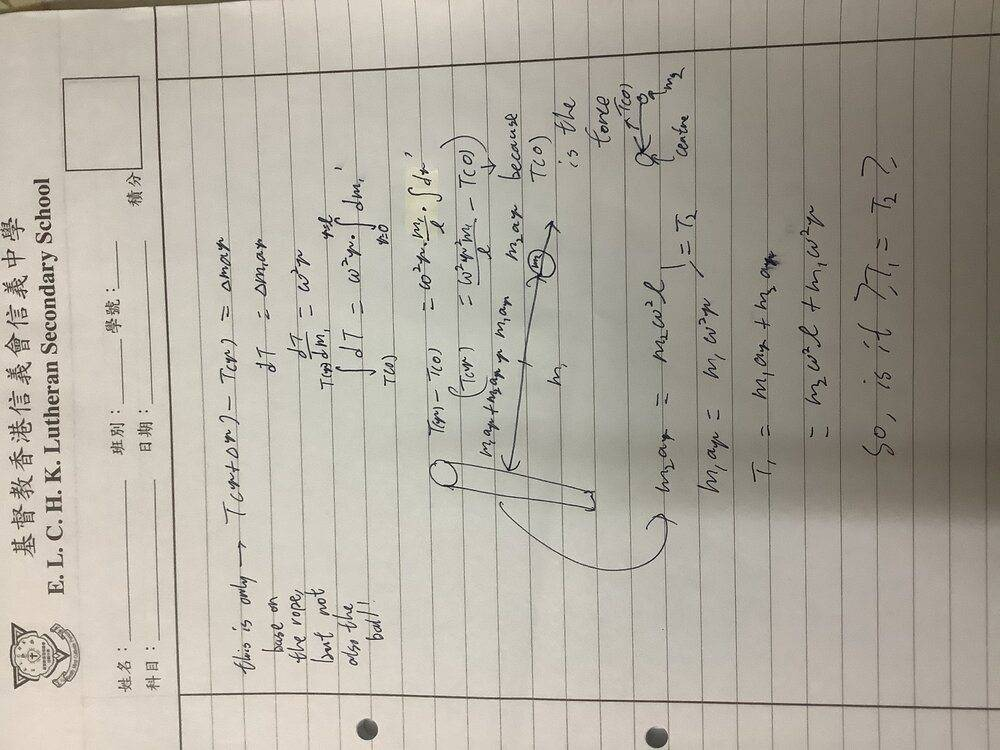𝑎𝑟

Because of object two, I am confusing about how to put the tension affect by the object to combine the tension on the rope.It is the tension at r=L is m_2*omega^2*L?

What I am wrong? Does there any hint for T(0), because T(L)is m_2*omega^2*L, but ... how about T(0) , I still can’t understand how to find it, as I don’t know is the relation of T(r), that it should not be 0.

But this is correct ? 𝑇(𝑟+Δ𝑟)−𝑇(𝑟)=Δ𝑚*a*r, I am getting confused on the relation
between T(0) and T(r), is it the x-direction acceleration should be 0?Is it T(0)-T(r)=0?
This is what I am confused the most.
View attachment 268416𝑎𝑟
You have ##dT=\omega^2r.dm_1##, which you turned into an integral as ##T(r)-T(0)=\int_0^r\omega^2r.dm_1##. That is not really a valid way to write it. The variable of integration is the thing after the 'd', so in your integral it is ##m_1##, but the bounds should refer to that variable, whereas your bounds refer to r.
You then wrote it as ##\omega^2r\int.dm_1##, but ##dm_1=\rho.dr##, where ##\rho## is the linear density of the rope. You cannot move outside the integral terms which vary through the integral.
You need to solve ##T(r)-T(0)=\int_0^r\omega^2r\rho .dr##

Does there any hint for T(0), because T(L)is m_2*omega^2*L
Use the known value at L to deduce T(0).
And please learn to use latex.

I got ## { m_1 \omega^2 {( l^2 - 1)} / {l}} ## = T(r)
It is I should add back ## m_2 \omega^2 l ## ?
I had use two different method result two different answer, but I think there are some mistake, but I am still a beginner of calculus, so can you tell me where I state wrong?
Second answer: ## {{ 1 - r / l } * {m_1 \omega^2}} + m_2 \omega^2 l##
I find myself typing in something wrong, this time is the correct one.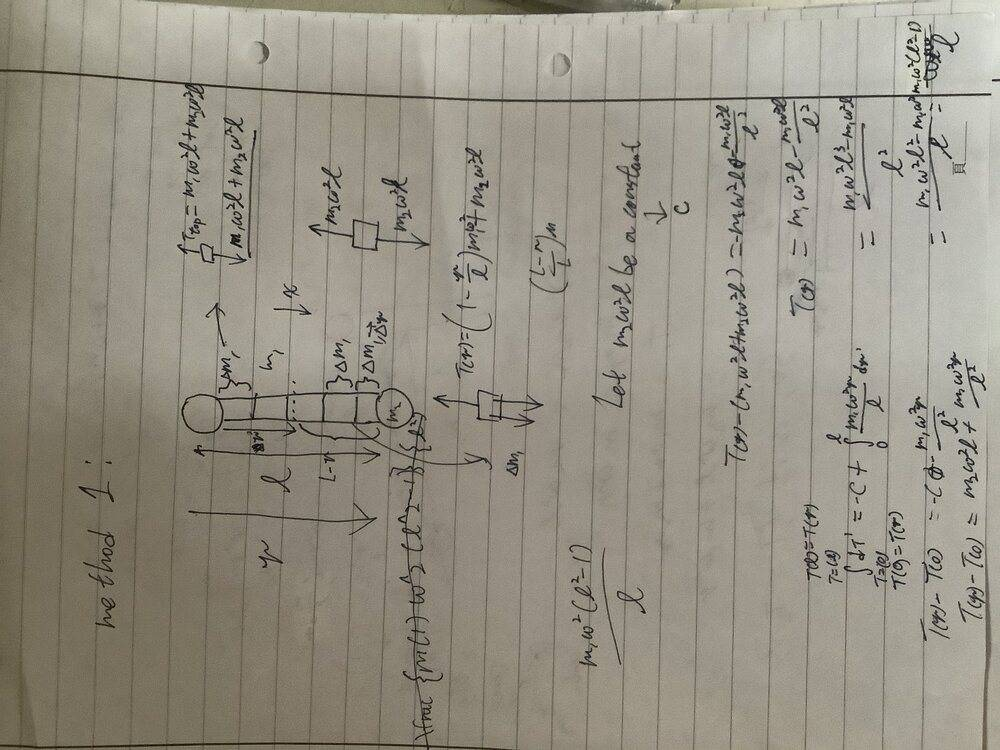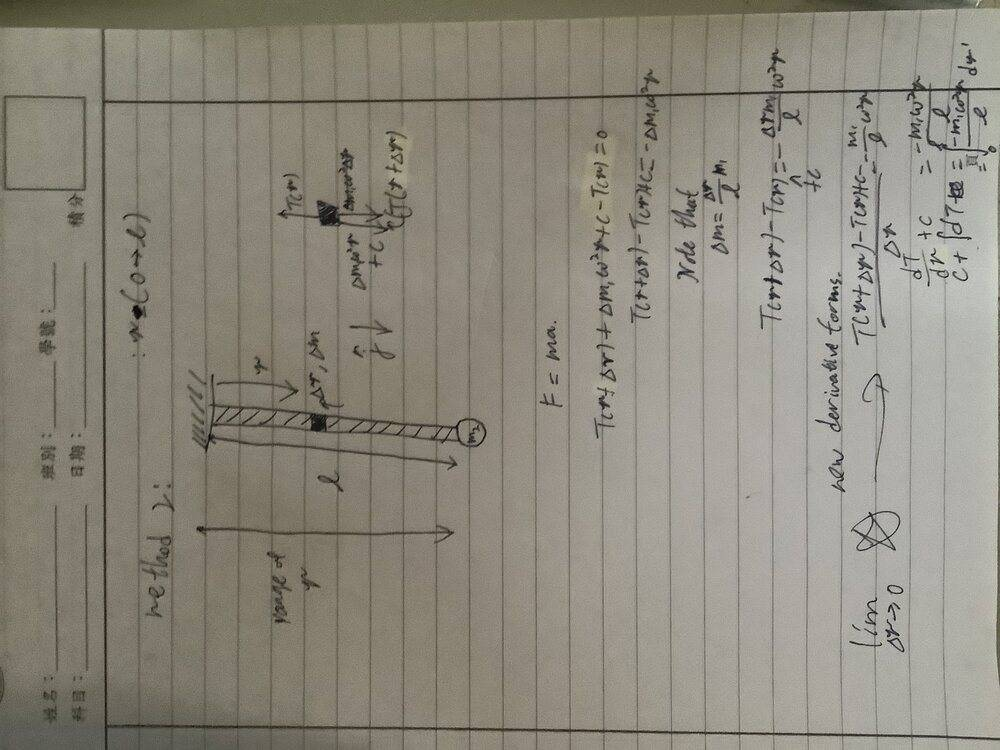Last edited:
I got ## { m_1 \omega^2 { l^2 - 1} / {l^2 - 1}} ## = T(r)
You should realize this is impossible since it is dimensionally inconsistent. In dimensional terms this reads MT-2L2 - L-2 -1 = MLT-2.
All terms added, subtracted or compared should be dimensionally the same.
You can use this check to work through your algebra to see where the mistake(s) occurred. This is a hugely useful technique.

If you want me to find your mistake, post it all as typed in, preferably in Latex. Per forum guidelines, images are intended for diagrams and textbook extracts, not for handwritten algebra.

I got a new answer, this maybe correct now!(maybe...)

## c = m_2 \omega^2 l##, because l is a constant so ,the force c will become a constant.
##(lim(dr=0))T( r + dr) + dm_1##
How are you defining c?

You had error in your latex ('{' and '}' instead of '(' and ')'). Use the Preview button to check what your latex says.

Even then, I have no idea what ##(lim(dr=0))T( r + dr) + dm_1## means. Should there be an equals sign in there?

Before you can find T(0) you have to get the right expression for T(r)-T(0). I told you in post #13 your integration is wrong.
First, try to get your integration right, then plug in r=L to get the expression it gives for T(L)-T(0), then plug in the known value of T(L).

•MichaelTam
I got a new answer, this maybe correct now!(maybe...)

##c=m_2 ω^2 l##, because l is a constant so ,the force c will become a constant and m_2 also a constant and \omega also a constant
##T {r+T dr} + dm_1 ω^2 r − Tr + c =0##
Note that ##dm_1=(m_1/l)∗dr##

And then I get ## {dT+c} /dr=−m1ω2r/l##, the (dT + c )/dr

then...##T{r}= m_1 \omega^2 r - {m_1 \omega^2 r^2}/l ##
sorry, I don’t know why I can’t convert them into Latex now.
After repost ,I can do it now.
or, it is need to add the c back?
Because the ##m_2 \omega^2 l## is also affect the T(r)...

Last edited:
I retreat the integration to
## dT+c =-{ dr m_1 \omega^2 r} / l ##
Is it correct?
Should I remove the constant out the integration , after integrates it?
And the density in this question is also supposed to be balance in the rope.(same I mean.)
It is T(r)-T(0) is not equal to 0?
I still don’t know the real expression of T(r) and T(0) , i am finding ways to express them, but I still can’t sure they are right,
it is T(0) should be = ##m_2 \omega^2 l + m_1 \omega^2 r##
If it is right , I will get ##- 2 m_1 \omega^2 r = T{r}##

Last edited:
I retreat the integration to
## dT+c =-{ dr m_1 \omega^2 r} / l ##
Is it correct?
No, it does not even make sense as an equation.
dT and dr are infinitesimals, but c is not, so you cannot add c to those. You add in the constant of integration as part of the integration step, not beforehand.
But if you use the form ##T(r)-T(0)=\int_0^r...## you do not need a constant of integration.
And you are still not integrating ## \int dr .m_1 \omega^2 r/ l ## correctly. What is ##\int x.dx##?

Equal x^2/2 + c?Sorry, but it is quite difficult to type in Latex, but my problem is very urgent.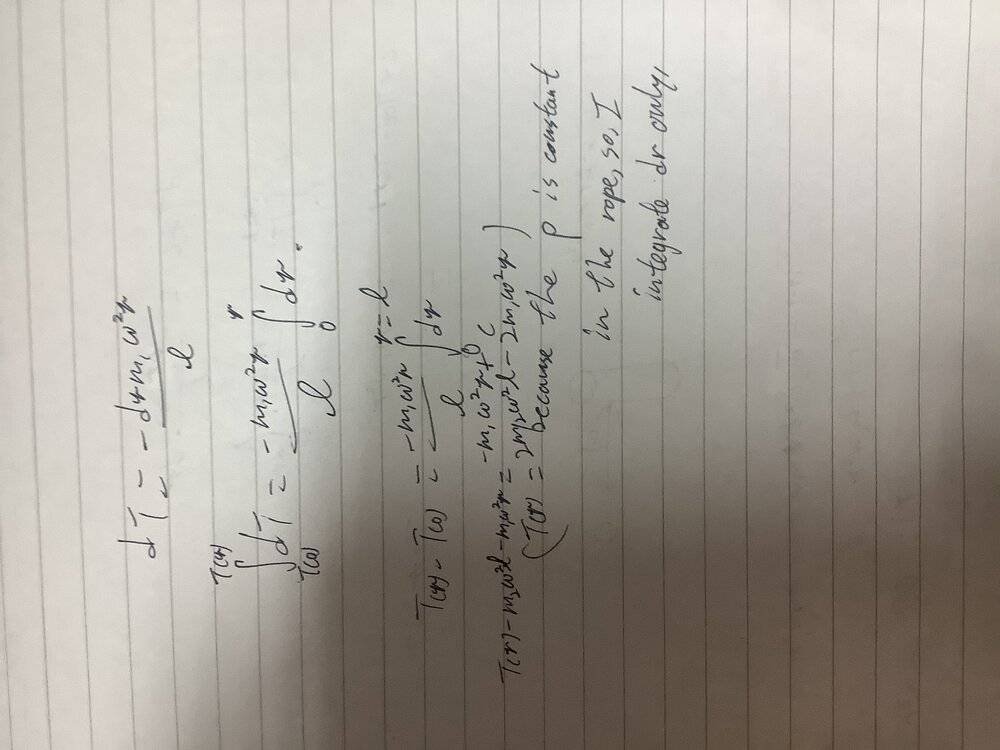Equal x^2/2 + c?Sorry, but it is quite difficult to type in Latex, but my problem is very urgent.
We will make better progress if you try to understand what I am telling you. It seems that if I write something you do not understand you just ignore it.
## \int dr .m_1 \omega^2 r/ l ## is not ## m_1 \omega^2 r/ l \int dr##. You can take the mass, l, and ω outside because they are constants, but that leaves you with ## m_1 \omega^2 /l \int _0^r rdr##. Can you see why I asked you how to integrate ##\int x.dx##?

I didn’t ignore your suggestion, I just trying to understand to step to reach the solution, and I am sorry ,maybe I am doing a little bit too hurry that I had forget some principal.

And I want to thank you, that Tension in Rope Wrapped Around a Rod, I got the correct answer finally!Thank you very much!

I don’t know why my answer is still incorrect

I don’t know why my answer is still incorrect
You got the right answer for ##\int x.dx##, so why can't you apply the same rule to ##\int r.dr##?

Is it I should do like this?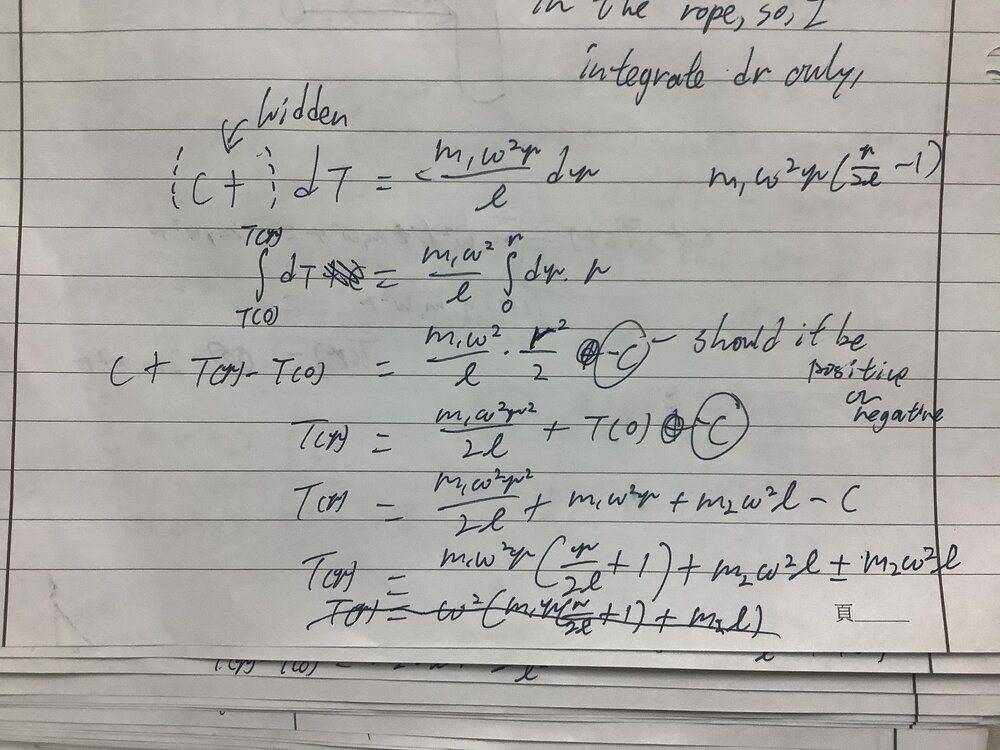Last edited:
Is it I should do like this?View attachment 268456
Better, but you are ignoring what I told you in post #20.
If you have an integral equation ##\int_{t_0}^{t}dt=\int _{r_0}^{r}2r.dr## you can write ##\Delta t=\Delta r^2## or ##t-t_0=r^2-r_0^2## or ##t=r^2+c##. You do not need to write ##\Delta t=\Delta r^2+c##.
In the ##t=r^2+c## form, you can substitute the boundary values to get ##t_0=r_0^2+c## to find that ##c=t_0-r_0^2##, so ##t=r^2+t_0-r_0^2##, exactly the same as with the other forms.

##\lambda = \frac{m_1}{l} = \frac{\Delta{m}}{\Delta{x}}## linear density

I got ##\int \limits_x^l \, dT=\int \limits_x^l \, -\lambda \omega^2 x dx\Rightarrow T(x)=T(l) + \lambda \omega^2 \frac{l^2-x^2}{2}##

##T(l)=m_2 \omega^2 l \Rightarrow T(x) = \omega^2 \big(m_2 l + m_1 \frac{l^2-x^2}{2l} \big) ## final answer

This gud?

Last edited:
##\lambda = \frac{m_1}{l} = \frac{\Delta{m}}{\Delta{x}}## linear density

I got ##\int \limits_x^l \, dT=\int \limits_x^l \, -\lambda \omega^2 x dx\Rightarrow T(x)=T(l) + \lambda \omega^2 \frac{l^2-x^2}{2}##

##T(l)=m_2 \omega^2 l \Rightarrow T(x) = \omega^2 \big(m_2 l + m_1 \frac{l^2-x^2}{2l} \big) ## final answer

This gud?
Yes.

You have ##dT=\omega^2r.dm_1##, which you turned into an integral as ##T(r)-T(0)=\int_0^r\omega^2r.dm_1##. That is not really a valid way to write it. The variable of integration is the thing after the 'd', so in your integral it is ##m_1##, but the bounds should refer to that variable, whereas your bounds refer to r.
You then wrote it as ##\omega^2r\int.dm_1##, but ##dm_1=\rho.dr##, where ##\rho## is the linear density of the rope. You cannot move outside the integral terms which vary through the integral.
You need to solve ##T(r)-T(0)=\int_0^r\omega^2r\rho .dr##
For this portion of the problem, I managed to get T(r)-T(0) =(m_1* omega^2 * r^2)/2l

For this portion of the problem, I managed to get T(r)-T(0) =(m_1* omega^2 * r^2)/2l
Looks right. Looks even better with proper use of parentheses, dropping the redundant "*"s, adding the bracing pairs of "#" and "\" in front of "omega":
##T(r)-T(0) =(m_1\omega^2 r^2)/(2l)##

•AzimD
Looks right. Looks even better with proper use of parentheses, dropping the redundant "*"s, adding the bracing pairs of "#" and "\" in front of "omega":
##T(r)-T(0) =(m_1\omega^2 r^2)/(2l)##
Thanks for checking my answer! Yep, I recently found the LaTeX Guide! I'll be attempting to use it from here on out.

Looks right. Looks even better with proper use of parentheses, dropping the redundant "*"s, adding the bracing pairs of "#" and "\" in front of "omega":
##T(r)-T(0) =(m_1\omega^2 r^2)/(2l)##
So going from here then: ##T(r)=m_1\omega^2(\frac{r^2}{2l} -l)##

Then considering the Tension caused by the ball ##T_b=-m_2\omega^2l##

We can combine the two tensions and get the actual T(r) to be ##T(r)=m_1\omega^2(\frac{r^2}{2l} -l) - m_2\omega^2l##

Am I conceptualizing this right? Or does there seem to be an error in my thought process? Am I making it to simple?

So going from here then: ##T(r)=m_1\omega^2(\frac{r^2}{2l} -l)##
Looking back through the thread, unless I misunderstand how the variables are defined, the equation of mine you quoted in post #31 is wrong. It has a sign error.
See if you can correct it.
(I believe T(0) should be the tension at the pole if we ignore the point mass.)

Also, what do you have for T(0)?

•erobz